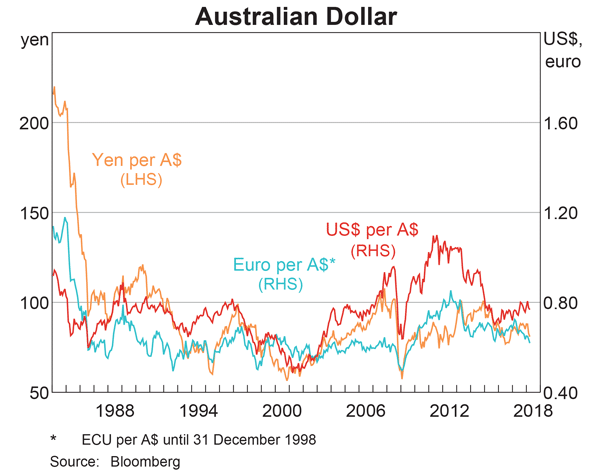# Fight Finance

#### CoursesTagsRandomAllRecentScores

The following equation is the Dividend Discount Model, also known as the 'Gordon Growth Model' or the 'Perpetuity with growth' equation.

$$P_{0} = \frac{C_1}{r_{\text{eff}} - g_{\text{eff}}}$$

What would you call the expression $C_1/P_0$?

 Portfolio Details Stock Expected return Standard deviation Correlation Dollars invested A 0.1 0.4 0.5 60 B 0.2 0.6 140

What is the expected return of the above portfolio?

A zero coupon bond that matures in 6 months has a face value of $1,000. The firm that issued this bond is trying to forecast its income statement for the year. It needs to calculate the interest expense of the bond this year. The bond is highly illiquid and hasn't traded on the market. But the finance department have assessed the bond's fair value to be$950 and this is its book value right now at the start of the year.

Assume that:

• the firm uses the 'effective interest method' to calculate interest expense.
• the market value of the bond is the same as the book value.
• the firm is only interested in this bond's interest expense. Do not include the interest expense for a new bond issued to refinance the current one, as would normally happen.

What will be the interest expense of the bond this year for the purpose of forecasting the income statement?

The following equation is the Dividend Discount Model, also known as the 'Gordon Growth Model' or the 'Perpetuity with growth' equation.

$$p_0=\frac{d_1}{r_\text{eff}-g_\text{eff}}$$

Which expression is NOT equal to the expected capital return?

Which one of the following bonds is trading at a discount?

 Project Data Project life 2 yrs Initial investment in equipment $600k Depreciation of equipment per year$250k Expected sale price of equipment at end of project $200k Revenue per job$12k Variable cost per job $4k Quantity of jobs per year 120 Fixed costs per year, paid at the end of each year$100k Interest expense in first year (at t=1) $16.091k Interest expense in second year (at t=2)$9.711k Tax rate 30% Government treasury bond yield 5% Bank loan debt yield 6% Levered cost of equity 12.5% Market portfolio return 10% Beta of assets 1.24 Beta of levered equity 1.5 Firm's and project's debt-to-equity ratio 25%

Notes

1. The project will require an immediate purchase of \$50k of inventory, which will all be sold at cost when the project ends. Current liabilities are negligible so they can be ignored.

Assumptions

• The debt-to-equity ratio will be kept constant throughout the life of the project. The amount of interest expense at the end of each period has been correctly calculated to maintain this constant debt-to-equity ratio. Note that interest expense is different in each year.
• Thousands are represented by 'k' (kilo).
• All cash flows occur at the start or end of the year as appropriate, not in the middle or throughout the year.
• All rates and cash flows are nominal. The inflation rate is 2% pa.
• All rates are given as effective annual rates.
• The 50% capital gains tax discount is not available since the project is undertaken by a firm, not an individual.

What is the net present value (NPV) of the project?

Question 432  option, option intrinsic value, no explanation

An American call option with a strike price of $K$ dollars will mature in $T$ years. The underlying asset has a price of $S$ dollars.

What is an expression for the current intrinsic value in dollars from owning (being long) the American call option? Note that the intrinsic value of an option does not subtract the premium paid to buy the option.

Which one of the below option and futures contracts gives the possibility of potentially unlimited gains?

Which one of the following capital raisings or payouts involve the sale of shares to existing shareholders only?

Examine the graph of the AUD versus the USD, EUR and JPY. Note that RHS means right hand side and LHS left hand side which indicates which axis each line corresponds to. Assume inflation rates in each country were equal over the time period 1984 to 2018.Which of the following statements is NOT correct?